4.8 Deletion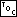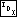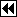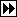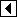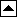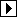Deleting an item from a binary search tree is a little harder than inserting one. Before we write any code, let's consider how to delete nodes from a binary search tree in an abstract fashion. Here's a BST from which we can draw examples during the discussion:

It is more difficult to remove some nodes from this tree than to remove others. Here, we recognize three distinct cases (Exercise 4.8-1 offers a fourth), described in detail below in terms of the deletion of a node designated p.

##### Case 1: p has no right child

It is trivial to delete a node with no right child, such as node 1, 4, 7, or 8 above. We replace the pointer leading to p by p's left child, if it has one, or by a null pointer, if not. In other words, we replace the deleted node by its left child. For example, the process of deleting node 8 looks like this: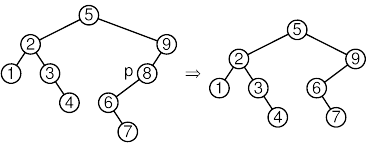##### Case 2: p's right child has no left child

This case deletes any node p with a right child r that itself has no left child. Nodes 2, 3, and 6 in the tree above are examples. In this case, we move r into p's place, attaching p's former left subtree, if any, as the new left subtree of r. For instance, to delete node 2 in the tree above, we can replace it by its right child 3, giving node 2's left child 1 to node 3 as its new left child. The process looks like this: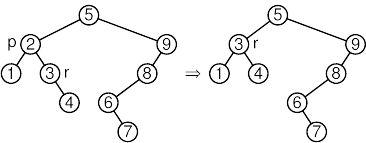##### Case 3: p's right child has a left child

This is the “hard” case, where p's right child r has a left child. but if we approach it properly we can make it make sense. Let p's inorder successor (see inorder successor), that is, the node with the smallest value greater than p, be s. Then, our strategy is to detach s from its position in the tree, which is always an easy thing to do, and put it into the spot formerly occupied by p, which disappears from the tree. In our example, to delete node 5, we move inorder successor node 6 into its place, like this: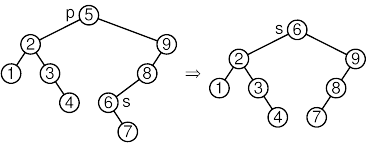But how do we know that node s exists and that we can delete it easily? We know that it exists because otherwise this would be case 1 or case 2 (consider their conditions). We can easily detach from its position for a more subtle reason: s is the inorder successor of p and is therefore has the smallest value in p's right subtree, so s cannot have a left child. (If it did, then this left child would have a smaller value than s, so it, rather than s, would be p's inorder successor.) Because s doesn't have a left child, we can simply replace it by its right child, if any. This is the mirror image of case 1.

##### Implementation

The code for BST deletion closely follows the above discussion. Let's start with an outline of the function:

```37. <BST item deletion function 37> =
void *bst_delete (struct bst_table *tree, const void *item) {
struct bst_node *p, *q; /* Node to delete and its parent. */
int cmp;                /* Comparison between p->bst_data and item. */
int dir;                /* Side of q on which p is located. */

assert (tree != NULL && item != NULL);

<Step 1: Find BST node to delete 38>
<Step 2: Delete BST node 39>
<Step 3: Finish up after deleting BST node 44>
}
```

This code is included in 29.

We begin by finding the node to delete, in much the same way that bst_find() did. But, in every case above, we replace the link leading to the node being deleted by another node or a null pointer. To do so, we have to keep track of the pointer that led to the node to be deleted. This is the purpose of q and dir in the code below.

```38. <Step 1: Find BST node to delete 38> =
p = (struct bst_node *) &tree->bst_root;
for (cmp = -1; cmp != 0;      cmp = tree->bst_compare (item, p->bst_data, tree->bst_param))   {
dir = cmp > 0;
q = p;
if (p == NULL)
return NULL;
}
item = p->bst_data;
```

This code is included in 37.

Now we can actually delete the node. Here is the code to distinguish between the three cases:

```39. <Step 2: Delete BST node 39> =
if (p->bst_link == NULL) { <Case 1 in BST deletion 40> }
else   {
struct bst_node *r = p->bst_link;
if (r->bst_link == NULL)       {
<Case 2 in BST deletion 41>
}     else       {
<Case 3 in BST deletion 42>
}
}
```

This code is included in 37.

In case 1, we simply replace the node by its left subtree:

```40. <Case 1 in BST deletion 40> =
```

This code is included in 39.

In case 2, we attach the node's left subtree as its right child r's left subtree, then replace the node by r:

```41. <Case 2 in BST deletion 41> =
```

This code is included in 39.

We begin case 3 by finding p's inorder successor as s, and the parent of s as r. Node p's inorder successor is the smallest value in p's right subtree and that the smallest value in a tree can be found by descending to the left until a node with no left child is found:

```42. <Case 3 in BST deletion 42> =
struct bst_node *s;
for (;;)   {
if (s->bst_link == NULL)
break;

r = s;
}
```

This code is included in 39.

Case 3 wraps up by adjusting pointers so that s moves into p's place:

```43. <Case 3 in BST deletion 42> +=
```

As the final step, we decrement the number of nodes in the tree, free the node, and return its data:

```44. <Step 3: Finish up after deleting BST node 44> =
tree->bst_alloc->libavl_free (tree->bst_alloc, p);
tree->bst_count–;
tree->bst_generation++;
return (void *) item;
```

This code is included in 37.

See also:  [Knuth 1998b], algorithm 6.2.2D; [Cormen 1990], section 13.3.

Exercises:

1. Write code for a case 1.5 which handles deletion of nodes with no left child. [answer]

2. In the code presented above for case 3, we update pointers to move s into p's position, then free p. An alternate approach is to replace p's data by s's and delete s. Write code to use this approach. Can a similar modification be made to either of the other cases? [answer]

*3. The code in the previous exercise is a few lines shorter than that in the main text, so it would seem to be preferable. Explain why the revised code, and other code based on the same idea, cannot be used in libavl. (Hint: consider the semantics of libavl traversers.) [answer]